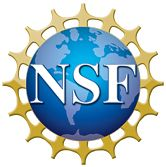ARCHIVED CONTENT: In December 2020, the CZO program was succeeded by the Critical Zone Collaborative Network (CZ Net) ×

## Perrot et al., 2014

#### Abstract

This study compares stream nitrate ( math formula) concentrations to spatially distributed snowmelt in two alpine catchments, the Green Lakes Valley, Colorado (GLV4) and Tokopah Basin, California (TOK). A snow water equivalent reconstruction model and Landsat 5 and 7 snow cover data were used to estimate daily snowmelt at 30 m spatial resolution in order to derive indices of new snowmelt areas (NSAs). Estimates of NSA were then used to explain the  math formula flushing behavior for each basin over a 12 year period (1996–2007). To identify the optimal method for defining NSAs and elucidate mechanisms underlying catchment  math formula flushing, we conducted a series of regression analyses using multiple thresholds of snowmelt based on temporal and volumetric metrics. NSA indices defined by volume of snowmelt (e.g., snowmelt ≤ 30 cm) rather than snowmelt duration (e.g., snowmelt ≤ 9 days) were the best predictors of stream  math formula concentrations. The NSA indices were better correlated with stream  math formula concentration in TOK (average R2= 0.68) versus GLV4 (average R2= 0.44). Positive relationships between NSA and stream  math formula concentration were observed in TOK with peak stream  math formula concentration occurring on the rising limb of snowmelt. Positive and negative relationships between NSA and stream  math formula concentration were found in GLV4 with peak stream  math formula concentration occurring as NSA expands. Consistent with previous works, the contrasting  math formula flushing behavior suggests that streamflow in TOK was primarily influenced by overland flow and shallow subsurface flow, whereas GLV4 appeared to be more strongly influenced by deeper subsurface flow paths.

#### Citation

Perrot, D., N. P. Molotch, M. W. Williams, S. M. Jepsen, and J. O. Sickman (2014): Relationships between stream nitrate concentration and spatially distributed snowmelt in high-elevation catchments of the western US. Water Resour. Res., 50, 8694–8713. DOI: 10.1002/2013WR015243This Paper/Book acknowledges NSF CZO grant support.